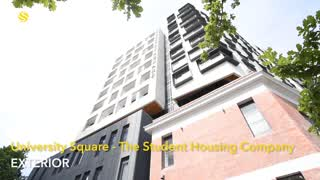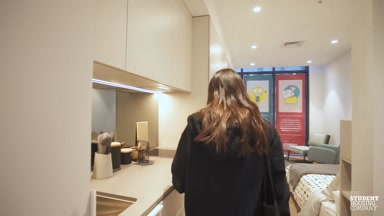# University Square学生公寓查看全部照片
A\$319 /周

• Semester 2开放预订
• 接受18岁以下未成年人
• 高速网络不限流量
• 免费健身房

1226 位学生已收藏此公寓

## 安家离墨尔本大学和皇家墨尔本理工大学咫尺之距的University Square，坐拥当地各色咖啡店及集市

• 地址:

85 Barry Street, 墨尔本, 3053

• 前往皇家墨尔本理工大学：
• 15分钟
• 11分钟
• 6分钟
• 前往墨尔本大学：
• 17分钟
• 13分钟
• 7分钟
• 设施: 提供家具, 洗衣房
• 房租包含: 无线网, 水费, 电费
• ## 设施&服务

设施
• 电影放映室
• 自行车车库
• 公寓经理
• 电梯
• 娱乐区/室
• 图书馆/学习区
• 提供家具
• 公共洗衣房（投币式）
安全&保障
• 闭路电视/监控摄像机
• 维修团队（24小时待命）
• 安全门禁（大门上锁）
公寓规定
• 禁止吸烟
• 公共区域禁酒
公寓规定
• 禁止吸烟
• 公共区域禁酒
公寓规定
• 禁止吸烟
• 公共区域禁酒
公寓规定
• 禁止吸烟
• 公共区域禁酒
公寓规定
• 禁止吸烟
• 公共区域禁酒
公寓规定
• 禁止吸烟
• 公共区域禁酒
公寓规定
• 禁止吸烟
• 公共区域禁酒
公寓规定
• 禁止吸烟
• 公共区域禁酒
公寓规定
• 禁止吸烟
• 公共区域禁酒
公寓规定
• 禁止吸烟
• 公共区域禁酒
公寓规定
• 禁止吸烟
• 公共区域禁酒
公寓规定
• 禁止吸烟
• 公共区域禁酒
公寓规定
• 禁止吸烟
• 公共区域禁酒
公寓规定
• 禁止吸烟
• 公共区域禁酒
公寓规定
• 禁止吸烟
• 公共区域禁酒
公寓规定
• 禁止吸烟
• 公共区域禁酒
公寓规定
• 禁止吸烟
• 公共区域禁酒
公寓规定
• 禁止吸烟
• 公共区域禁酒
公寓规定
• 禁止吸烟
• 公共区域禁酒
公寓规定
• 禁止吸烟
• 公共区域禁酒
公寓规定
• 禁止吸烟
• 公共区域禁酒
公寓规定
• 禁止吸烟
• 公共区域禁酒
公寓规定
• 禁止吸烟
• 公共区域禁酒
公寓规定
• 禁止吸烟
• 公共区域禁酒
公寓规定
• 禁止吸烟
• 公共区域禁酒
公寓规定
• 禁止吸烟
• 公共区域禁酒
公寓规定
• 禁止吸烟
• 公共区域禁酒
公寓规定
• 禁止吸烟
• 公共区域禁酒
公寓规定
• 禁止吸烟
• 公共区域禁酒
公寓规定
• 禁止吸烟
• 公共区域禁酒
公寓规定
• 禁止吸烟
• 公共区域禁酒
公寓规定
• 禁止吸烟
• 公共区域禁酒
公寓规定
• 禁止吸烟
• 公共区域禁酒
公寓规定
• 禁止吸烟
• 公共区域禁酒
公寓规定
• 禁止吸烟
• 公共区域禁酒
公寓规定
• 禁止吸烟
• 公共区域禁酒
公寓规定
• 禁止吸烟
• 公共区域禁酒
公寓规定
• 禁止吸烟
• 公共区域禁酒
公寓规定
• 禁止吸烟
• 公共区域禁酒
公寓规定
• 禁止吸烟
• 公共区域禁酒
公寓规定
• 禁止吸烟
• 公共区域禁酒
公寓规定
• 禁止吸烟
• 公共区域禁酒
公寓规定
• 禁止吸烟
• 公共区域禁酒
公寓规定
• 禁止吸烟
• 公共区域禁酒
公寓规定
• 禁止吸烟
• 公共区域禁酒
公寓规定
• 禁止吸烟
• 公共区域禁酒
公寓规定
• 禁止吸烟
• 公共区域禁酒
公寓规定
• 禁止吸烟
• 公共区域禁酒
公寓规定
• 禁止吸烟
• 公共区域禁酒
公寓规定
• 禁止吸烟
• 公共区域禁酒
公寓规定
• 禁止吸烟
• 公共区域禁酒
公寓规定
• 禁止吸烟
• 公共区域禁酒
公寓规定
• 禁止吸烟
• 公共区域禁酒
公寓规定
• 禁止吸烟
• 公共区域禁酒
公寓规定
• 禁止吸烟
• 公共区域禁酒
公寓规定
• 禁止吸烟
• 公共区域禁酒
公寓规定
• 禁止吸烟
• 公共区域禁酒
公寓规定
• 禁止吸烟
• 公共区域禁酒
公寓规定
• 禁止吸烟
• 公共区域禁酒
公寓规定
• 禁止吸烟
• 公共区域禁酒
公寓规定
• 禁止吸烟
• 公共区域禁酒
公寓规定
• 禁止吸烟
• 公共区域禁酒
公寓规定
• 禁止吸烟
• 公共区域禁酒
公寓规定
• 禁止吸烟
• 公共区域禁酒
公寓规定
• 禁止吸烟
• 公共区域禁酒
公寓规定
• 禁止吸烟
• 公共区域禁酒
公寓规定
• 禁止吸烟
• 公共区域禁酒
公寓规定
• 禁止吸烟
• 公共区域禁酒
公寓规定
• 禁止吸烟
• 公共区域禁酒
公寓规定
• 禁止吸烟
• 公共区域禁酒
公寓规定
• 禁止吸烟
• 公共区域禁酒
公寓规定
• 禁止吸烟
• 公共区域禁酒
公寓规定
• 禁止吸烟
• 公共区域禁酒
公寓规定
• 禁止吸烟
• 公共区域禁酒
公寓规定
• 禁止吸烟
• 公共区域禁酒
公寓规定
• 禁止吸烟
• 公共区域禁酒
公寓规定
• 禁止吸烟
• 公共区域禁酒
公寓规定
• 禁止吸烟
• 公共区域禁酒
公寓规定
• 禁止吸烟
• 公共区域禁酒
公寓规定
• 禁止吸烟
• 公共区域禁酒
公寓规定
• 禁止吸烟
• 公共区域禁酒
公寓规定
• 禁止吸烟
• 公共区域禁酒
公寓规定
• 禁止吸烟
• 公共区域禁酒
公寓规定
• 禁止吸烟
• 公共区域禁酒
公寓规定
• 禁止吸烟
• 公共区域禁酒
公寓规定
• 禁止吸烟
• 公共区域禁酒
公寓规定
• 禁止吸烟
• 公共区域禁酒
公寓规定
• 禁止吸烟
• 公共区域禁酒
公寓规定
• 禁止吸烟
• 公共区域禁酒
公寓规定
• 禁止吸烟
• 公共区域禁酒
公寓规定
• 禁止吸烟
• 公共区域禁酒
公寓规定
• 禁止吸烟
• 公共区域禁酒
公寓规定
• 禁止吸烟
• 公共区域禁酒
公寓规定
• 禁止吸烟
• 公共区域禁酒
公寓规定
• 禁止吸烟
• 公共区域禁酒
公寓规定
• 禁止吸烟
• 公共区域禁酒
公寓规定
• 禁止吸烟
• 公共区域禁酒
公寓规定
• 禁止吸烟
• 公共区域禁酒
公寓规定
• 禁止吸烟
• 公共区域禁酒
公寓规定
• 禁止吸烟
• 公共区域禁酒
公寓规定
• 禁止吸烟
• 公共区域禁酒
公寓规定
• 禁止吸烟
• 公共区域禁酒
公寓规定
• 禁止吸烟
• 公共区域禁酒
公寓规定
• 禁止吸烟
• 公共区域禁酒
公寓规定
• 禁止吸烟
• 公共区域禁酒
公寓规定
• 禁止吸烟
• 公共区域禁酒
公寓规定
• 禁止吸烟
• 公共区域禁酒
公寓规定
• 禁止吸烟
• 公共区域禁酒
公寓规定
• 禁止吸烟
• 公共区域禁酒
公寓规定
• 禁止吸烟
• 公共区域禁酒
公寓规定
• 禁止吸烟
• 公共区域禁酒
公寓规定
• 禁止吸烟
• 公共区域禁酒
公寓规定
• 禁止吸烟
• 公共区域禁酒
公寓规定
• 禁止吸烟
• 公共区域禁酒
公寓规定
• 禁止吸烟
• 公共区域禁酒
公寓规定
• 禁止吸烟
• 公共区域禁酒
公寓规定
• 禁止吸烟
• 公共区域禁酒
公寓规定
• 禁止吸烟
• 公共区域禁酒
公寓规定
• 禁止吸烟
• 公共区域禁酒
公寓规定
• 禁止吸烟
• 公共区域禁酒
公寓规定
• 禁止吸烟
• 公共区域禁酒
公寓规定
• 禁止吸烟
• 公共区域禁酒
公寓规定
• 禁止吸烟
• 公共区域禁酒
公寓规定
• 禁止吸烟
• 公共区域禁酒
公寓规定
• 禁止吸烟
• 公共区域禁酒
公寓规定
• 禁止吸烟
• 公共区域禁酒
公寓规定
• 禁止吸烟
• 公共区域禁酒
公寓规定
• 禁止吸烟
• 公共区域禁酒
公寓规定
• 禁止吸烟
• 公共区域禁酒
公寓规定
• 禁止吸烟
• 公共区域禁酒
公寓规定
• 禁止吸烟
• 公共区域禁酒
公寓规定
• 禁止吸烟
• 公共区域禁酒
公寓规定
• 禁止吸烟
• 公共区域禁酒
公寓规定
• 禁止吸烟
• 公共区域禁酒
公寓规定
• 禁止吸烟
• 公共区域禁酒
公寓规定
• 禁止吸烟
• 公共区域禁酒
公寓规定
• 禁止吸烟
• 公共区域禁酒
公寓规定
• 禁止吸烟
• 公共区域禁酒
公寓规定
• 禁止吸烟
• 公共区域禁酒
公寓规定
• 禁止吸烟
• 公共区域禁酒
公寓规定
• 禁止吸烟
• 公共区域禁酒
公寓规定
• 禁止吸烟
• 公共区域禁酒
公寓规定
• 禁止吸烟
• 公共区域禁酒
公寓规定
• 禁止吸烟
• 公共区域禁酒
公寓规定
• 禁止吸烟
• 公共区域禁酒
公寓规定
• 禁止吸烟
• 公共区域禁酒
公寓规定
• 禁止吸烟
• 公共区域禁酒
公寓规定
• 禁止吸烟
• 公共区域禁酒
公寓规定
• 禁止吸烟
• 公共区域禁酒
公寓规定
• 禁止吸烟
• 公共区域禁酒
公寓规定
• 禁止吸烟
• 公共区域禁酒
公寓规定
• 禁止吸烟
• 公共区域禁酒
公寓规定
• 禁止吸烟
• 公共区域禁酒
公寓规定
• 禁止吸烟
• 公共区域禁酒
公寓规定
• 禁止吸烟
• 公共区域禁酒
公寓规定
• 禁止吸烟
• 公共区域禁酒
公寓规定
• 禁止吸烟
• 公共区域禁酒
公寓规定
• 禁止吸烟
• 公共区域禁酒
公寓规定
• 禁止吸烟
• 公共区域禁酒
公寓规定
• 禁止吸烟
• 公共区域禁酒
公寓规定
• 禁止吸烟
• 公共区域禁酒
公寓规定
• 禁止吸烟
• 公共区域禁酒
公寓规定
• 禁止吸烟
• 公共区域禁酒
公寓规定
• 禁止吸烟
• 公共区域禁酒
公寓规定
• 禁止吸烟
• 公共区域禁酒
公寓规定
• 禁止吸烟
• 公共区域禁酒
公寓规定
• 禁止吸烟
• 公共区域禁酒
公寓规定
• 禁止吸烟
• 公共区域禁酒
公寓规定
• 禁止吸烟
• 公共区域禁酒
公寓规定
• 禁止吸烟
• 公共区域禁酒
公寓规定
• 禁止吸烟
• 公共区域禁酒
公寓规定
• 禁止吸烟
• 公共区域禁酒
公寓规定
• 禁止吸烟
• 公共区域禁酒
公寓规定
• 禁止吸烟
• 公共区域禁酒
公寓规定
• 禁止吸烟
• 公共区域禁酒
公寓规定
• 禁止吸烟
• 公共区域禁酒
公寓规定
• 禁止吸烟
• 公共区域禁酒
公寓规定
• 禁止吸烟
• 公共区域禁酒
公寓规定
• 禁止吸烟
• 公共区域禁酒
公寓规定
• 禁止吸烟
• 公共区域禁酒
公寓规定
• 禁止吸烟
• 公共区域禁酒
公寓规定
• 禁止吸烟
• 公共区域禁酒
公寓规定
• 禁止吸烟
• 公共区域禁酒
公寓规定
• 禁止吸烟
• 公共区域禁酒
公寓规定
• 禁止吸烟
• 公共区域禁酒
公寓规定
• 禁止吸烟
• 公共区域禁酒
公寓规定
• 禁止吸烟
• 公共区域禁酒
公寓规定
• 禁止吸烟
• 公共区域禁酒
公寓规定
• 禁止吸烟
• 公共区域禁酒
公寓规定
• 禁止吸烟
• 公共区域禁酒
公寓规定
• 禁止吸烟
• 公共区域禁酒
公寓规定
• 禁止吸烟
• 公共区域禁酒
公寓规定
• 禁止吸烟
• 公共区域禁酒
公寓规定
• 禁止吸烟
• 公共区域禁酒
公寓规定
• 禁止吸烟
• 公共区域禁酒
公寓规定
• 禁止吸烟
• 公共区域禁酒
公寓规定
• 禁止吸烟
• 公共区域禁酒
公寓规定
• 禁止吸烟
• 公共区域禁酒
公寓规定
• 禁止吸烟
• 公共区域禁酒
公寓规定
• 禁止吸烟
• 公共区域禁酒
公寓规定
• 禁止吸烟
• 公共区域禁酒
公寓规定
• 禁止吸烟
• 公共区域禁酒
公寓规定
• 禁止吸烟
• 公共区域禁酒
公寓规定
• 禁止吸烟
• 公共区域禁酒
公寓规定
• 禁止吸烟
• 公共区域禁酒
公寓规定
• 禁止吸烟
• 公共区域禁酒
公寓规定
• 禁止吸烟
• 公共区域禁酒
公寓规定
• 禁止吸烟
• 公共区域禁酒
公寓规定
• 禁止吸烟
• 公共区域禁酒
公寓规定
• 禁止吸烟
• 公共区域禁酒
公寓规定
• 禁止吸烟
• 公共区域禁酒
公寓规定
• 禁止吸烟
• 公共区域禁酒
公寓规定
• 禁止吸烟
• 公共区域禁酒
公寓规定
• 禁止吸烟
• 公共区域禁酒
公寓规定
• 禁止吸烟
• 公共区域禁酒
公寓规定
• 禁止吸烟
• 公共区域禁酒
公寓规定
• 禁止吸烟
• 公共区域禁酒
公寓规定
• 禁止吸烟
• 公共区域禁酒
公寓规定
• 禁止吸烟
• 公共区域禁酒
公寓规定
• 禁止吸烟
• 公共区域禁酒
公寓规定
• 禁止吸烟
• 公共区域禁酒
公寓规定
• 禁止吸烟
• 公共区域禁酒
公寓规定
• 禁止吸烟
• 公共区域禁酒
公寓规定
• 禁止吸烟
• 公共区域禁酒
公寓规定
• 禁止吸烟
• 公共区域禁酒
公寓规定
• 禁止吸烟
• 公共区域禁酒
公寓规定
• 禁止吸烟
• 公共区域禁酒
公寓规定
• 禁止吸烟
• 公共区域禁酒
公寓规定
• 禁止吸烟
• 公共区域禁酒
公寓规定
• 禁止吸烟
• 公共区域禁酒
公寓规定
• 禁止吸烟
• 公共区域禁酒
公寓规定
• 禁止吸烟
• 公共区域禁酒
公寓规定
• 禁止吸烟
• 公共区域禁酒
公寓规定
• 禁止吸烟
• 公共区域禁酒
公寓规定
• 禁止吸烟
• 公共区域禁酒
公寓规定
• 禁止吸烟
• 公共区域禁酒
公寓规定
• 禁止吸烟
• 公共区域禁酒
公寓规定
• 禁止吸烟
• 公共区域禁酒
公寓规定
• 禁止吸烟
• 公共区域禁酒
公寓规定
• 禁止吸烟
• 公共区域禁酒
公寓规定
• 禁止吸烟
• 公共区域禁酒
公寓规定
• 禁止吸烟
• 公共区域禁酒
公寓规定
• 禁止吸烟
• 公共区域禁酒
公寓规定
• 禁止吸烟
• 公共区域禁酒
公寓规定
• 禁止吸烟
• 公共区域禁酒
公寓规定
• 禁止吸烟
• 公共区域禁酒
公寓规定
• 禁止吸烟
• 公共区域禁酒
公寓规定
• 禁止吸烟
• 公共区域禁酒
公寓规定
• 禁止吸烟
• 公共区域禁酒
公寓规定
• 禁止吸烟
• 公共区域禁酒
公寓规定
• 禁止吸烟
• 公共区域禁酒
公寓规定
• 禁止吸烟
• 公共区域禁酒
公寓规定
• 禁止吸烟
• 公共区域禁酒
公寓规定
• 禁止吸烟
• 公共区域禁酒
公寓规定
• 禁止吸烟
• 公共区域禁酒
公寓规定
• 禁止吸烟
• 公共区域禁酒
公寓规定
• 禁止吸烟
• 公共区域禁酒
公寓规定
• 禁止吸烟
• 公共区域禁酒
公寓规定
• 禁止吸烟
• 公共区域禁酒
公寓规定
• 禁止吸烟
• 公共区域禁酒
公寓规定
• 禁止吸烟
• 公共区域禁酒

## 房租详情

全包式房租

此为最终租金价格，不加收其他费用

• 无线网（有限额）
• 水费
• 财产保险
• 电费
设施和费用以最终合同为准。
• #### 优质房源保障

甄选优质的海外学生公寓，提供安全舒适的住宿体验

• #### 优惠价格保证

找到更低价格，享受相同优惠价格

• #### 24小时专业顾问团队服务

订房顾问老师自身拥有留学经验，全天候为您提供专业的建议和服务